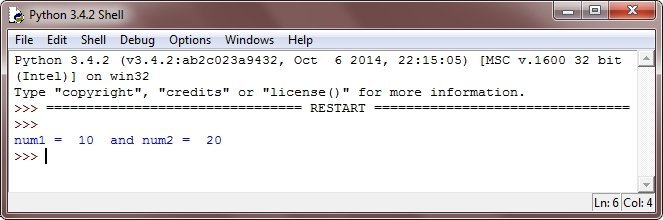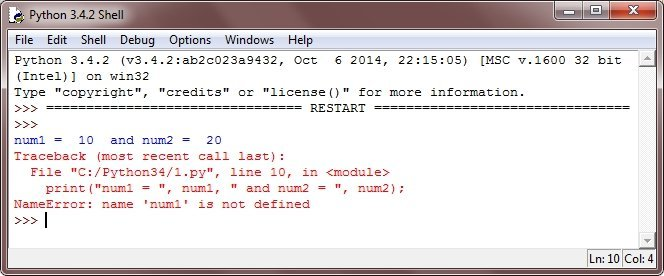# Python Numbers

Number data types are used to store numeric values in python program. Changing the value of a number data type results in a newly allocated object. Number object are simply created why you assign a value to them.

## Python Number Example

Here is an example of number data type in python

```# Python Number Data Type - Example Program

num1 = 10
num2 = 20

print("num1 = ", num1, " and num2 = ", num2);```

Here is the sample output of the above python program:## Delete the Reference to a Number Object in Python

To delete the reference to a number object in python, use the keyword del. Here is the general form to use del keyword in your python program:

`del var1[,var2[,var3[....,varN]]]]`

Here is the general form to delete a single object or multiple objects, using the keyword del in python:

```del var
del var1, var2```

### Example

Here is an example, illustrates the use of del keyword in python:

```# Python Number Data Type - Example Program

num1 = 10
num2 = 20

print("num1 = ", num1, " and num2 = ", num2);

del num1, num2

print("num1 = ", num1, " and num2 = ", num2);```

Here is the sample run of the above python program:Tools
Calculator